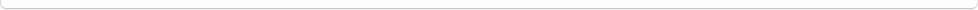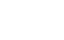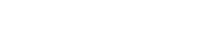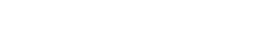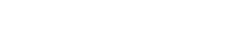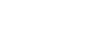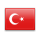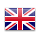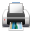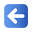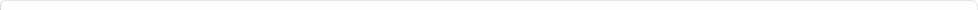Description of Individual Course Units
 Course Unit Code Course Unit Title Type of Course Unit Year of Study Semester Number of ECTS Credits 180104003100 DIFFERENTIAL EQUATIAONS Compulsory 2 3 5
Level of Course Unit
First Cycle
Objectives of the Course
to introduce different types of differential equations in engineering problems and, to investigate the solving process of them.
Name of Lecturer(s)
Dr.Öğr.Üyesi Melih YILDIZ
Learning Outcomes
 1 being able to define different type of differential equations 2 Being able to establish the differential equations of some physical problems 3 being able to solve the first order ordinary diferential equations, 4 being able to solve some differential equations with higher order ordinary diferential equations,
Mode of Delivery
Daytime Class
Prerequisites and co-requisities
no prerequisties
Recommended Optional Programme Components
to solve differantial equations requires a reasonable math background, therefore; it is strongly recommended that students review the basic topics such as dependent and independent variables, continuous and discontinuous functions, ordinary and partial derivates and, integrals before attending the course.
Course Contents
defining different types of differential equations and, solutions of 1th and high order differential equations in various ways.
Weekly Detailed Course Contents
 Week Theoretical Practice Laboratory 1 Definition and Classification of Differential Equations 2 Separable Differential Equations, Convertible Differential equations to separable form and, modeling 3 Exact Differential Equations,Convertible Differential equations to exact differential equation form (Integrating factor) 4 the first order linear differential equations and their solving ways(integrating factors and the method of variation of parameters 5 Reduction to Linear form -Bernoulli Equation 6 2th order differential equations-homogenous linear differential equations 7 Midterm Exam 8 Euler-Cauchy differential equations, Differential operators, existince -uniqueness theory and Wronskian 9 2th order non-homogeneous differential equations and solution methods( undetermined coefficients method and, the method of variation of parameters) 10 2th order non-homogeneous Euler-Cauchy differential equations and solution method( undetermined coefficients method and, the method of variation of parameters) 11 High order homogeneous and non-homogeneous differential equations and solution methods( undetermined coefficients method and, the method of variation of parameters) 12 High order homogeneous and non-homogeneous differential equations and solution methods( undetermined coefficients method and, the method of variation of parameters) 13 Solution of differential equations with Laplace Tranforms 14 Solution of differential equations with Laplace Tranforms 15 Final Exam
Erwin Kreyszig, "Advanced Engineering Mathematics", 9th edition,Waley Çengel, Y.A, Palm, W.J. Mühendisler ve Fen Bilimleri için Diferansiyel Denklemler,(Editor: Tahsin Engin),Güven Kitabevi, İzmir. Başarı, M.,Turker, E.S., Çözümlü Problemlerle Diferansiyel Denklemler, 2003, Değişim Yayınları.
Planned Learning Activities and Teaching Methods
Assessment Methods and Criteria
 Term (or Year) Learning Activities Quantity Weight Midterm Examination 1 100 SUM 100 End Of Term (or Year) Learning Activities Quantity Weight Final Examination 1 100 SUM 100 Term (or Year) Learning Activities 40 End Of Term (or Year) Learning Activities 60 SUM 100
Language of Instruction
Work Placement(s)